Latest Banking jobs   »

# Reasoning Ability Quiz For FCI Phase I 2022- 18th September

Direction (1-5): In this question, relationship between different elements is shown in the statements. The statements are followed by conclusions. Study the conclusions based on the given statement and select the appropriate answer.

Q1. Statements: A≥B=Q≤P<J≤Y; Z≥A>X; A<M
Conclusions: I. B<Y
II. X≥J
(a) Only conclusion I is true.
(b) Neither conclusion I nor II is true.
(c) Only conclusion II is true.
(d) Both conclusions I and conclusion II are true.
(e) Either conclusion I or II is true.

Q2. Statements: M>A≥B=Q; Z≥A>X; Q≤P<J≤Y
Conclusions: I. Z=Q
II. Z>Q
(a) Only conclusion I is true.
(b) Neither conclusion I nor II is true.
(c) Only conclusion II is true.
(d) Both conclusion I and conclusion II are true.
(e) Either conclusion I or II is true.

Q3. Statements: T<R; X<G<R=A≤S
Conclusions: I. G<S
II. S>T
(a) Only conclusion I is true.
(b) Neither conclusion I nor II is true.
(c) Only conclusion II is true.
(d) Both conclusions I and conclusion II are true.
(e) Either conclusion I or II is true.

Q4. Statements: M<K≤I>N; D≥P; I≥C; P=U<M
Conclusions: I. M<C
II. N>U
(a) Only conclusion I is true.
(b) Neither conclusion I nor II is true.
(c) Only conclusion II is true.
(d) Both conclusions I and conclusion II are true.
(e) Either conclusion I or II is true.

Q5. Statements: P=U<M<K≤I>N; D≥P; I≥C
Conclusions: I. D≥K
II. I>P
(a) Only conclusion I is true.
(b) Neither conclusion I nor II is true.
(c) Only conclusion II is true.
(d) Both conclusions I and conclusion II are true.
(e) Either conclusion I or II is true.

Directions (6-10): Study the following information carefully and answer the given questions.
Eight Persons are sitting around a square table, four of them sit at four corners facing inside while four sits at the middle of the sides facing outside. B sits third to the left of D. H sits second to the left of B and G sits third to the left of H. F is an immediate neighbour of D. A sits third to the left of the one who sits second to the left of F. E does not sits at corner. C sits immediate right of B.

Q6. How many persons sit between D and E, when counted right of D?
(a) Three
(b) One
(c) None
(d) Two
(e) Four

Q7. Who among the following sits immediate right of H?
(a) E
(b) D
(c) A
(d) C
(e) B

Q8. Who among the following faces G?
(a) D
(b) C
(c) B
(d) H
(e) A

Q9. What is the position of E with respect of B?
(a) Immediate right
(b) Second to the right
(c) Immediate Left
(d) Third to the left
(e) None of these

Q10. Four of the following are alike in a certain way so form a group, which among the following does not belong to that group?
(a) C
(b) D
(c) A
(d) B
(e) G

Direction (11-15): Study the following information to answer the given questions:
In a certain code language
‘Writer poetry prize’ is written as ‘la ac dt’,
‘Writer creative today magical service’ is written as ‘ol pr rp ac tp’,
‘Real poetry creative today coffee’ is written as ‘tp dt rp ge hg’
‘Stress creative service’ is written as ‘rt tp pr’.

Q11. What is the code for ‘service’?
(a) ol
(b) rp
(c) pr
(d) ac
(e) tp

Q12. ‘Stress today creative magical’ could be coded as?
(a) tp rt rp ol
(b) rt ac rp ge
(c) dt rt rp ol
(d) rt ac pr ol
(e) None of these

Q13. ‘hg’ stands for?
(a) poetry
(b) creative
(c) coffee
(d) real
(e) Either ‘real’ or ‘coffee’

Q14. Which of the following may be the code for ‘keep creative prize’?
(a) tp la rt
(b) la dt ol
(c) lb la tp
(d) ge la ac
(e) None of these

Q15. ‘tp ol pr’ is the code for?
(a) today creative service
(b) stress creative service
(c) creative magical service
(d) Can’t be determined
(e) None of these

Solutions

S1. Ans. (a)
S2. Ans. (e)
S3. Ans. (d)
S4. Ans. (b)
S5. Ans. (c)

Solution (6-10):
Sol.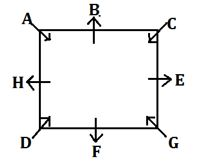S6. Ans. (d)
S7. Ans. (c)
S8. Ans. (e)
S9. Ans. (b)
S10. Ans. (d)

Solutions (11-15):
Sol.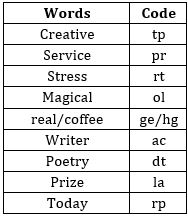S11. Ans. (c)
S12. Ans. (a)
S13. Ans. (e)
S14. Ans. (c)
S15. Ans. (c)#### Congratulations!Download Hindu Review of October 2021: Free PDFIncorrect details? Fill the form again here

Download Hindu Review of October 2021: Free PDF

Thank You, Your details have been submitted we will get back to you.
•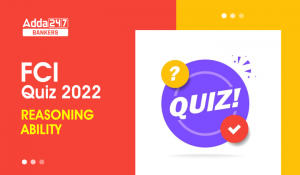Reasoning Ability Quiz For FCI Phase I 2...
•India to invest \$30 billion for 4G, 5G c...
•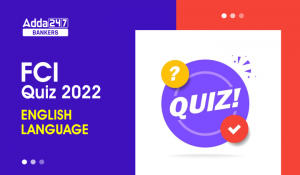English Quizzes For FCI Phase 1 2022- 26...
•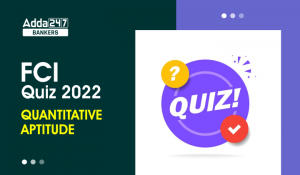Quantitative Aptitude Quiz For FCI Phase...
•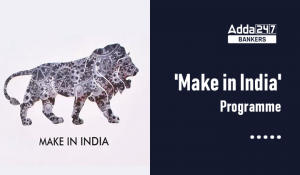Government's flagship programme 'Make in...
•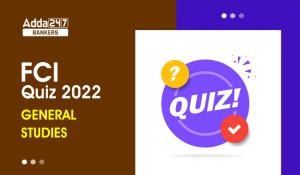General-Studies Quiz For FCI Phase I 202...